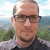# A Spatial View to calculate XY coordinates

4350
1
02-12-2016 02:17 AMOccasional Contributor II

I have a points layer with X,Y fields, each time I add a point I should perform "calculate geometry" to calculate the coordinates of the new points.

Is there a way to handle this automatically via a spatial view in SQL Server using a certain geometry function?

Any help is appreciated!byEsri Contributor

This is typically done using a SQL trigger that listens for incoming geometries either from editing a map document or inserting geometries with SQL.  When using a native DBMS spatial type such as SQL Server Geometry or Postgres' PostGIS type, constructor functions and spatial operator functions are provided that allow one to query different spatial properties.

For instance, in SQL Server we can use the

`shape.Lat, shape.Long`

functions for Geography types or

`shape.X and shape.Y`

for Geometry types on a spatial column called "shape".

Here is a simple SQL Server Geography example using shape.Lat and shape.Long. to update columns called "latitude" and "longitude" with their respective values.  This sample is provided AS IS and must be properly tested before implementing in a production environment.

``````CREATE TRIGGER [gis].[Insert_xy_if_geometry_fc] ON [gis].[Landmark_fc]
AFTER INSERT
,UPDATE
AS
UPDATE pt
SET pt.latitude = i.Shape.Lat
,pt.longitude = i.Shape.Long
FROM Landmark_fc AS pt
INNER JOIN (
SELECT objectid
,Shape
FROM inserted
WHERE (Shape IS NOT NULL)
) AS i ON i.objectid = pt.objectid;
RETURN
GO
‍‍‍‍‍‍‍‍‍‍‍‍‍‍‍‍‍‍‍‍‍‍‍‍‍‍‍‍‍‍‍‍‍``````

Lat (geography Data Type)

Long (geography Data Type)